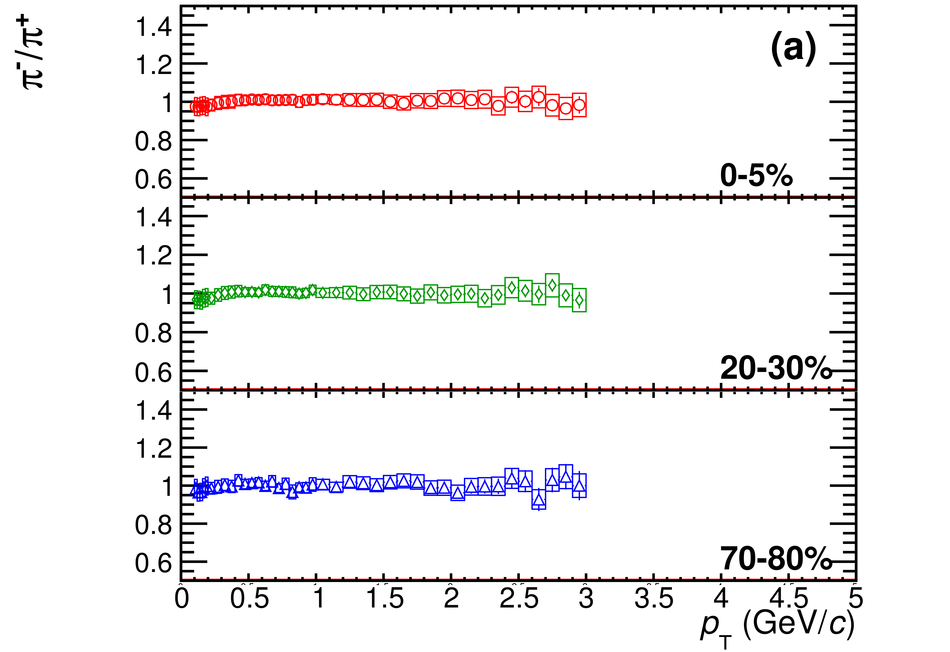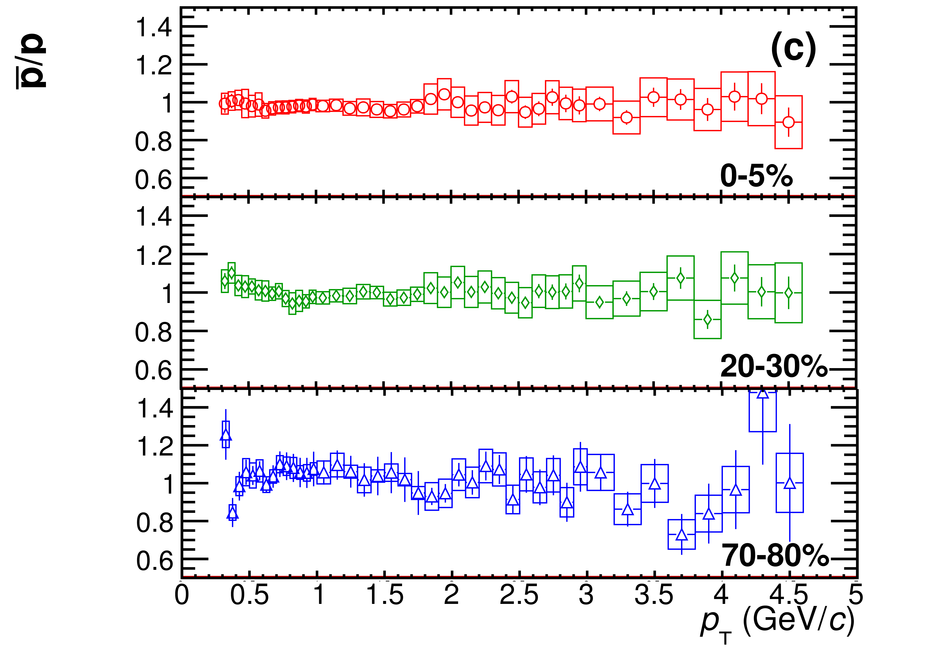# Figure 5

 (a) $\pi^-/\pi^+$ (b) $K^-/K^+$ (c) $\bar{\rm p}/{\rm p}$ ratios as a function of $\pt$ for central, semi-central and peripheral events.